Next: Dynamic Programming Up: Computational Methods in Finance Previous: Expected Values

# Duality and Qualitative Properties

The forward evolution equation () and the backward equation () are connected through a duality relation. For any time t, we compute () as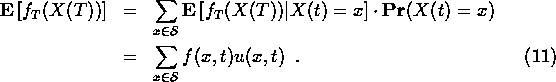For now, the main point is that the sum on the bottom line does not depend on t. Given the constancy of this sum and the u evolution equation (4), we can give another derivation of the f evolution equation (7). Start with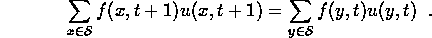Then use (4) on the left side and rearrange the sum: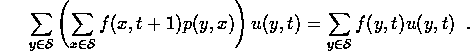Now, if this is going to be true for any u(y,t), the coefficients of u(y,t) on the left and right sides must be equal for each y. This gives (7). Similarly, it is possible to derive (4) from (7) and the constancy of the expected value.

The evolution equations (4) and (7) have some qualitative properties in common. The main one being that they preserve positivity. If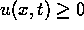for all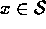, then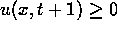for allalso. Likewise, if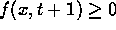for all x, then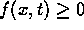for all x. These properties are simple consequences of (4) and (7) and the positivity of the p(x,y). Positivity preservation does not work in reverse. It is possible, for example, that f(x,t+1) ;SPMlt; 0 for some x even thoughfor all x.

The probability evolution equation (4) has a conservation law not shared by (7). It isindependent of t. This is natural if u is a probability distribution, so that the constant is 1. The expected value evolution equation (7) has a maximum principle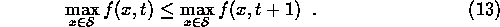This is a natural consequence of the interpretation of f as an expectation value. The probabilities, u(x,t) need not satisfy a maximum principle either forward of backward in time.

This duality relation has is particularly transparent in matrix terms. The formula () is expressed explicitly in terms of the probabilities at time t as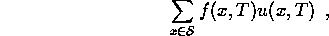which has the matrix form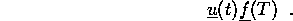Written in this order, the matrix multiplication is compatible; the other order,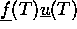, would represent an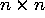matrix instead of a single number. In view of (), we may rewrite this as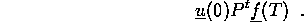Because matrix multiplication is associative, this may be rewritten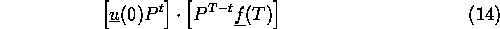for any t. This is the same as saying that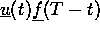is independent of t, as we already saw.

In linear algebra and functional analysis, ``adjoint'' or ``dual'' is a fancy generalization of the transpose operation of matrices. People who don't like to think of putting the vector to the left of the matrix think of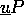as multiplication of (the transpose of)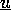, on the right, by the transpose (or adjoint or dual) of P. In other words, we can do enough evolution to compute an expected value either using P its dual (or adjoint or transpose). This is the origin of the term ``duality'' in this context.Next: Dynamic Programming Up: Computational Methods in Finance Previous: Expected Values

Jonathan Goodman
Tue Sep 15 17:12:32 EDT 1998